# Prime Factorization

Prime Factorization
Go back to  'Numbers'

Prime factorization is a way of expressing a number as a product of its prime factors.

Let’s take the example of the number 30

We know that 30 is 5 × 6, but 6 is not a prime number.

We also know that 6 is expressed as 2 × 3

Since 3 and 2 are prime numbers, the prime factorization of 30 is 2 × 3 × 5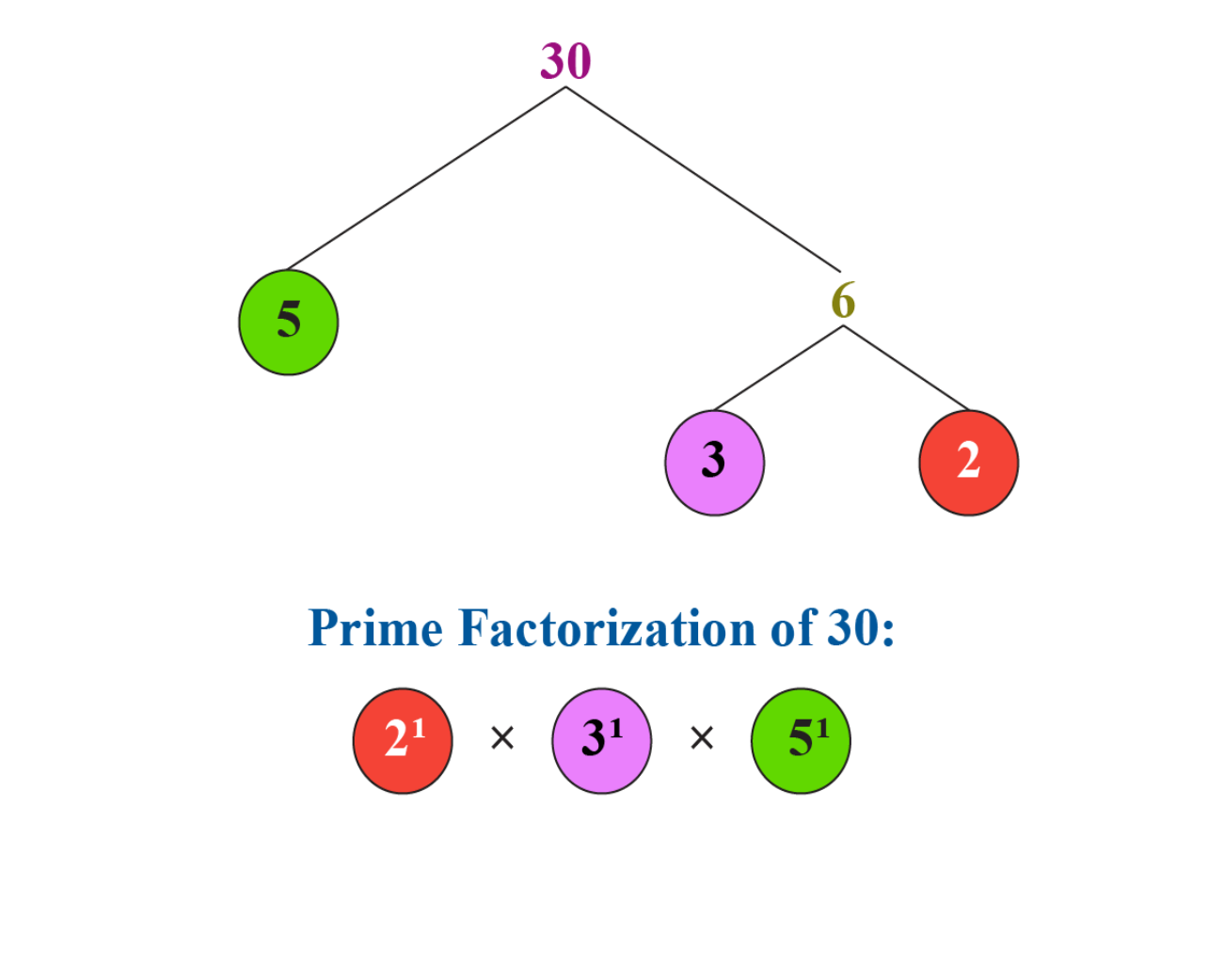Now that you have understood these basics, let us understand prime factorization better.

In this section, we will explore prime factorization, as well as the fundamental law of arithmetic using real-life examples.

Check-out the interactive simulation to know more about the lesson and try your hand at solving a few interesting practice questions on consecutive angles at the end of the page.

## Lesson Plan

 1 What Do You Mean by Prime Factorization? 2 Thinking Out of the Box! 3 Solved Examples on Prime Factorization 4 Challenging Questions on Prime Factorization 5 Interactive Questions on Prime Factorization

## What Do You Mean By Prime Factorization?

Prime factorization of any number means to represent that number as a product of prime numbers

For example, prime factorization of 40 is the representation of 40 as a product of prime numbers and can be done in the following way: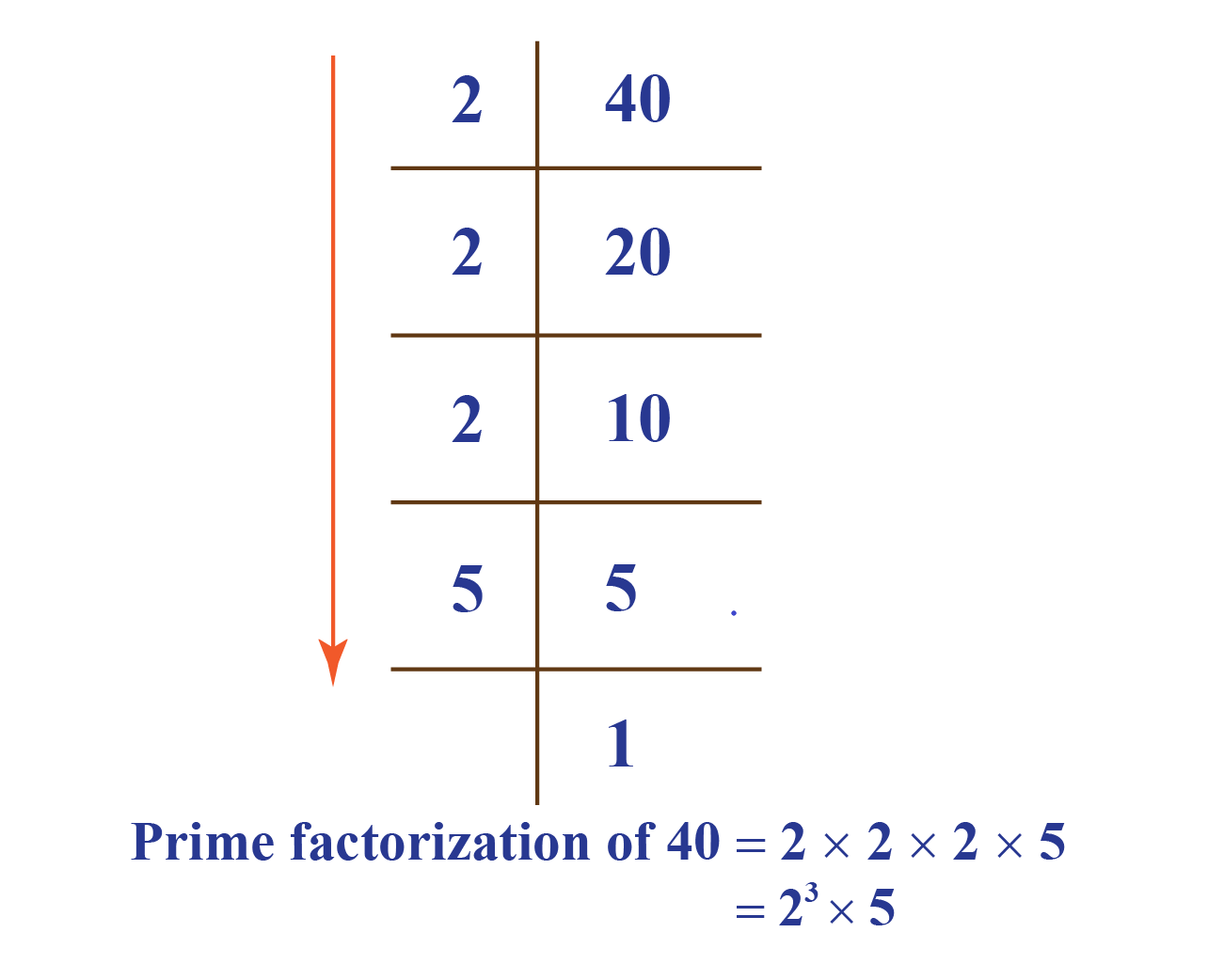Here,  2 and 5 are the prime numbers.

Let us see the prime factorization chart of a few more numbers in the following table:

Numbers Prime Factorization
36 $$2^2 \times 3^2$$
24 $$2^3 \times 3$$
60 $$2^2 \times 3 \times 5$$
18 $$2 \times 3^2$$
72 $$2^3 \times 3^2$$
45 $$3^2 \times 5$$
40 $$2^3 \times 5$$
50 $$2 \times 5^2$$
48 $$2^4 \times 3$$
30 $$2 \times 3 \times 5$$
42 $$2 \times 3 \times 7$$

## What Is the Fundamental Law of Arithmetic?

The statement of Fundamental Theorem Of Arithmetic is:

"Every composite number can be factorized as a product of primes, and this factorization is unique, apart from the order in which the prime factors occur."

For example, the prime factorization of $$240$$ is shown using a factor tree.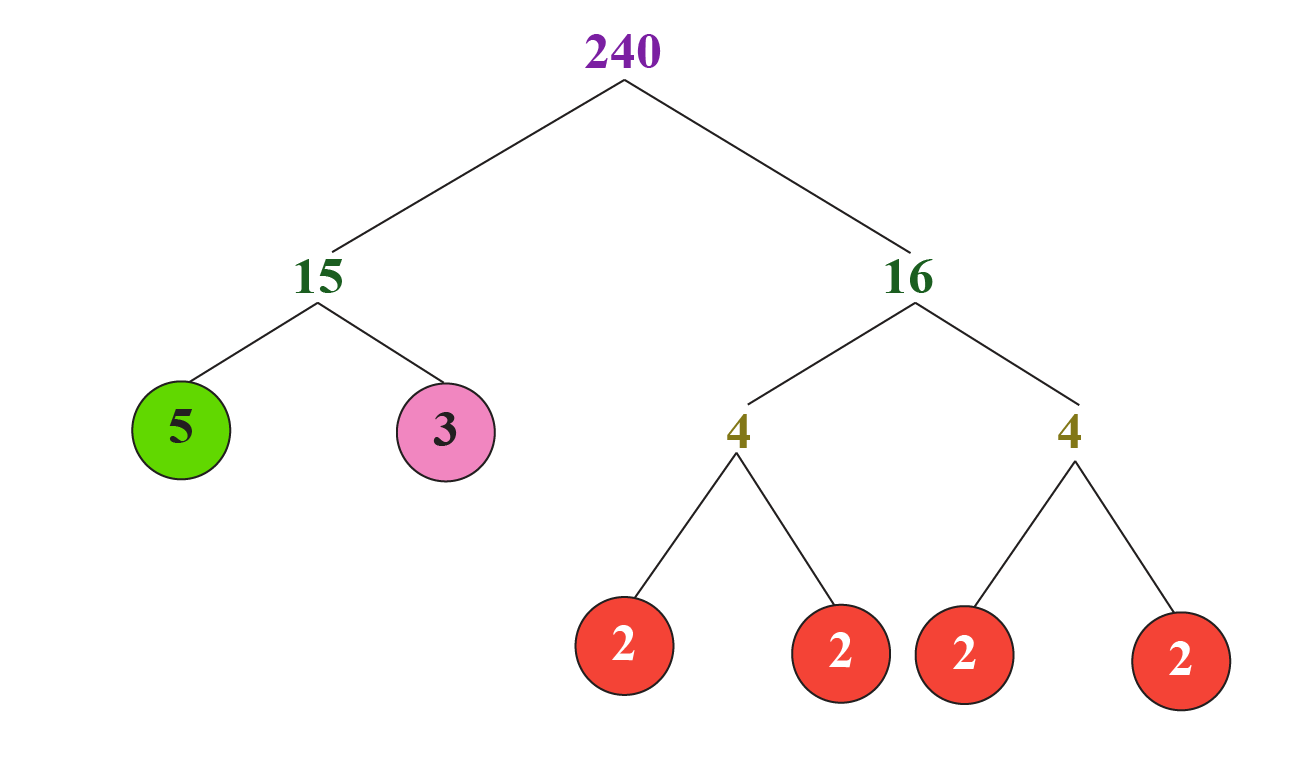From the above figure,\begin{aligned} 240 &=2 \times 2 \times 2 \times 2 \times 3 \times 5 \\ &=2^{4} \times 3^{1} \times 5^{1} \end{aligned}

Our theorem further tells us that this factorization must be unique.

That is, there is no other way to express $$240$$ as a product of primes.

Of course, we can change the order in which the prime factors occur.

For example, the prime factorization can be written as: \begin{aligned} 240 &=3^{1} \times 2^{4} \times 5^{1} \\ &=3^{1} \times 2^{2} \times 5^{1} \times 2^{2} \end{aligned}

But the set of prime factors (and the number of times each factor occurs) is unique.

That is, $$240$$ can have only one possible prime factorization, with four factors of $$2$$, one factor of $$3$$, and one factor of $$5$$

## Applications of Prime Factorization

### Cryptography

Cryptography is a method of protecting the information and communicating cryptography through the use of codes.

Prime factorization plays an important role for the coders who want to create a unique code using numbers that is not too heavy for computers to store or process quickly.

### HCF and LCM Using Fundamental Theorem of Arithmetic

To find the HCF and LCM of two numbers, we use the fundamental theorem of arithmetic.

For this, we first find the prime factorization of both the numbers.

Next, we consider the following:

• HCF is the product of the smallest power of each common prime factor.
• LCM is the product of the greatest power of each common prime factor.

Example:

What is the HCF of $$850$$ and $$680$$?

Solution:

We first find the prime factorizations of these numbers.

The prime factorization of 850 is shown below: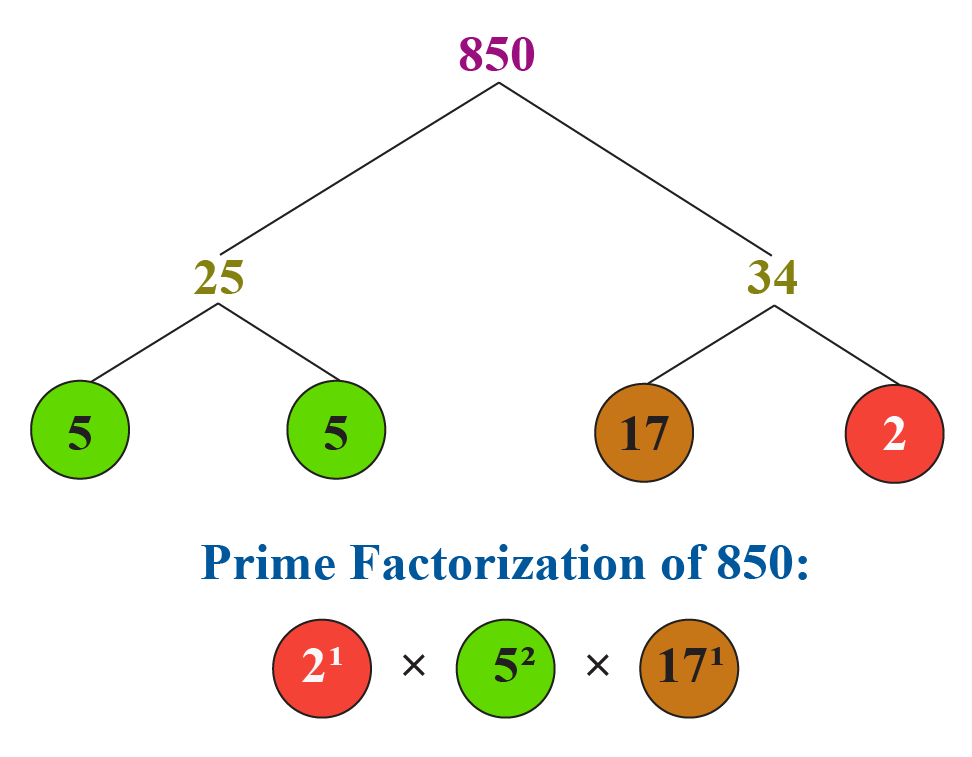The prime factorization of 680 is shown below: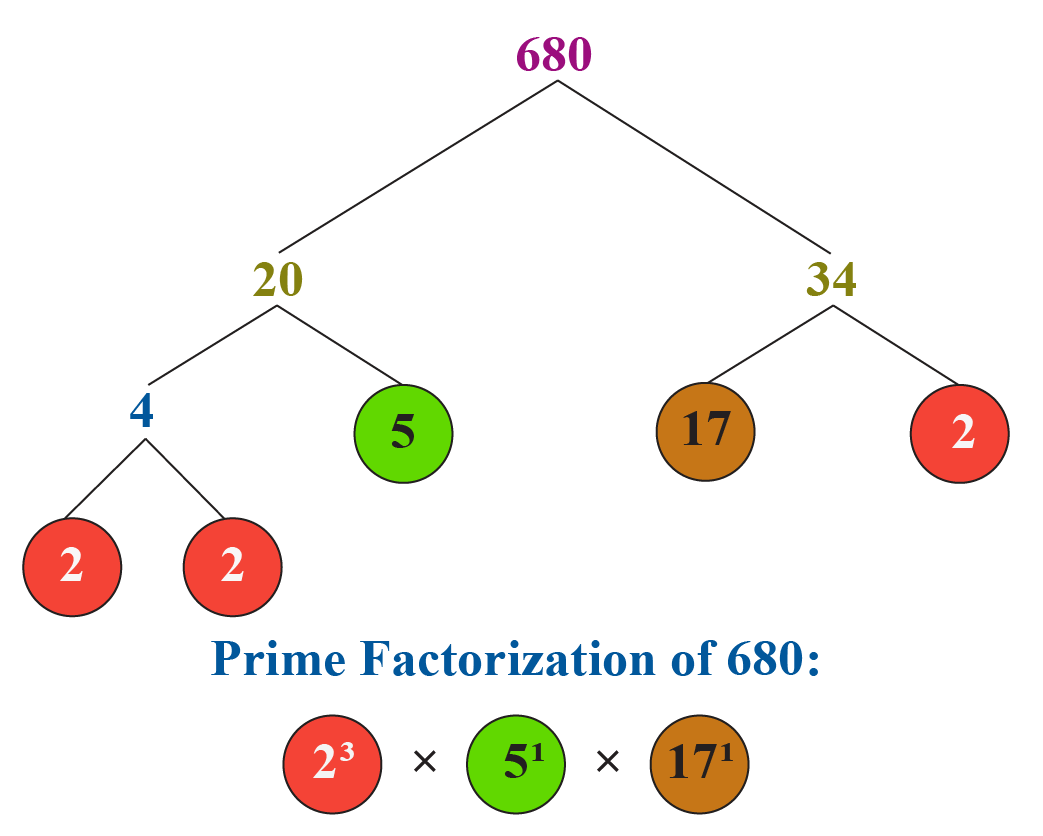Thus:

\begin{align} 850&=2^{1} \times 5^{2} \times 17^{1}\\[0.3cm]680&=2^{3} \times 5^{1} \times 17^{1} \end{align}

HCF is the product of the smallest power of each common prime factor.

Hence,

$\text{HCF }(850, 680) = 2^1 \times 5^1 \times 17^1 = 170$

LCM is the product of the greatest power of each common prime factor.

Hence,

$\text{LCM }(850, 680) = 2^3 \times 5^2 \times 17^1 = 3400$

Thus,

 $\text{HCF }(850, 680) = 170\\[0.3cm]\text{LCM }(850, 680) = 3400$Think Tank
• Suzy has to find the prime factorization of 24 using only the pair factors of 24 which has one odd number, and one even number in the factor pair. Can you help her do that?

## Solved Examples

 Example 1

Can you help Charlize to express $$1080$$ as the product of prime factors?

Also, can you tell if this factorization is unique?

Solution

We will find the prime factorization of $$1080$$: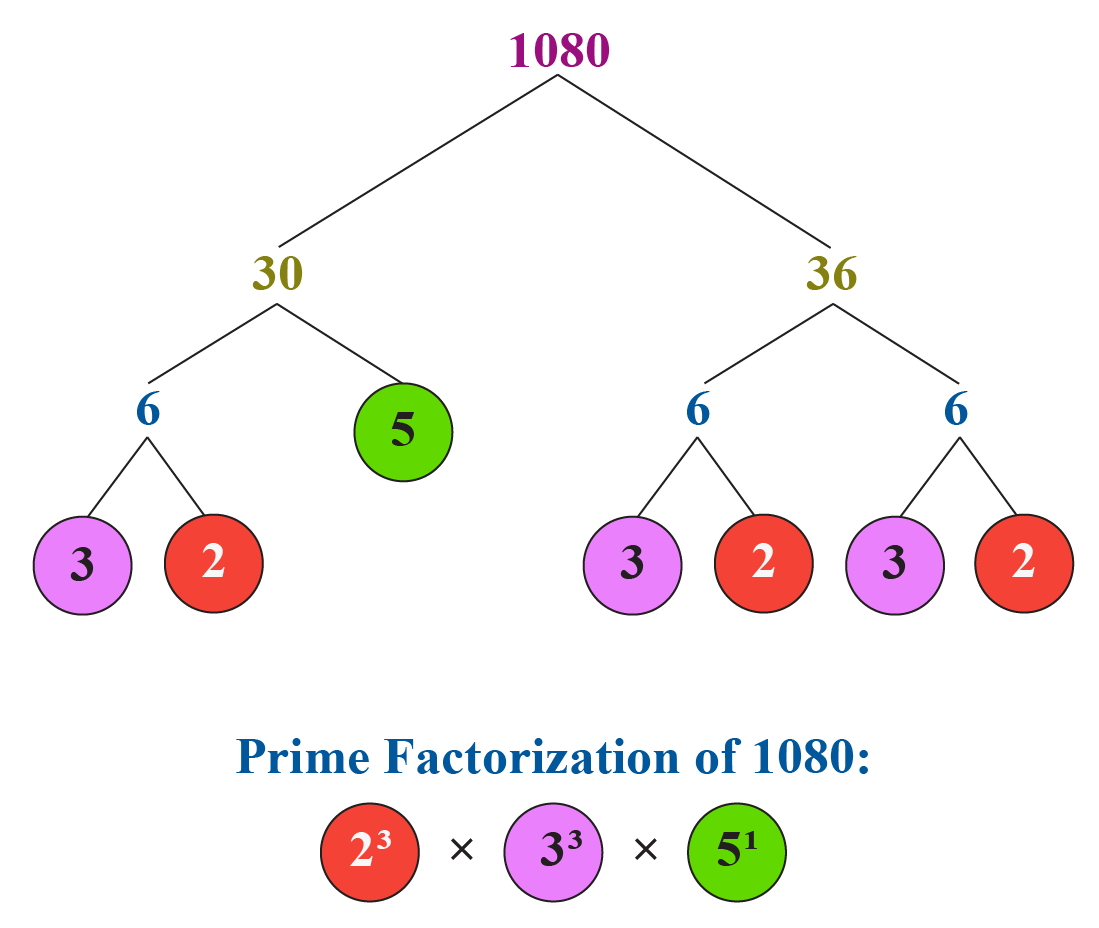The above prime factorization is unique by the fundamental theorem of arithmetic.

 Thus, $$1080=2^{3} \times 3^{3} \times 5^{1}$$
 Example 2

Charlot wants to find the HCF of $$126, 162$$ and $$180$$ using the fundamental theorem of arithmetic.

Can you help her find the answer to this problem?

Solution

We will find the prime factorizations of $$126, 162$$ and $$180$$

The prime factorization of 126 is shown below: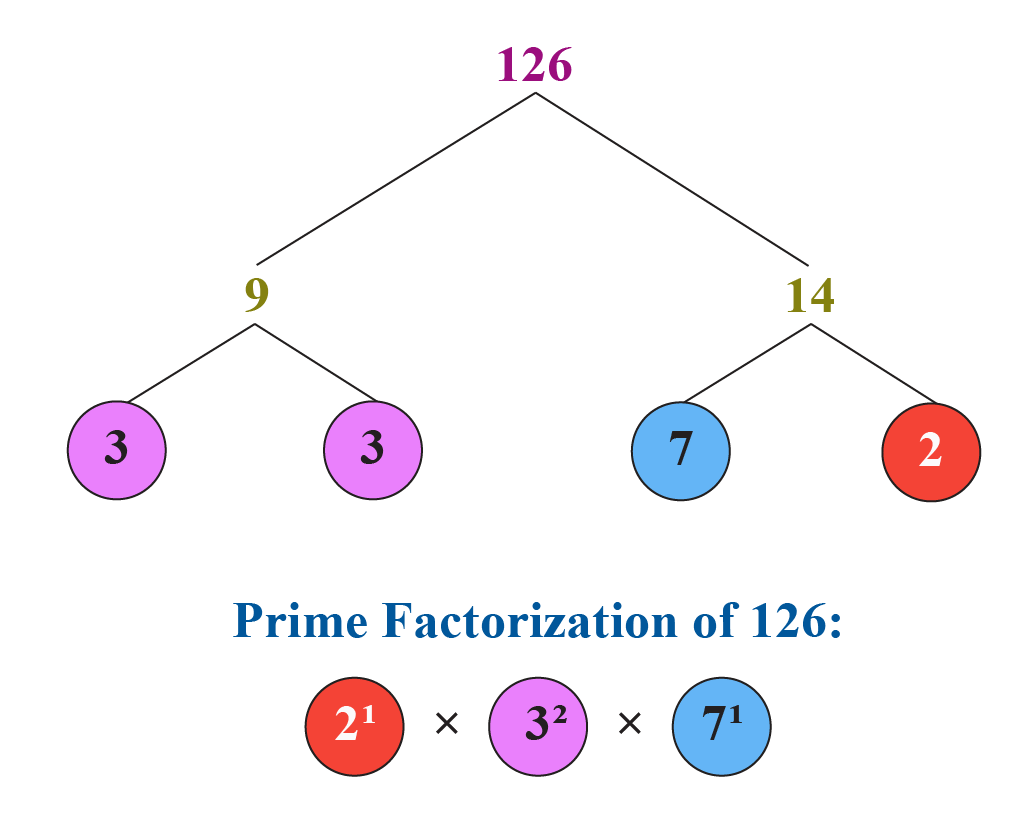The prime factorization of 162 is shown below: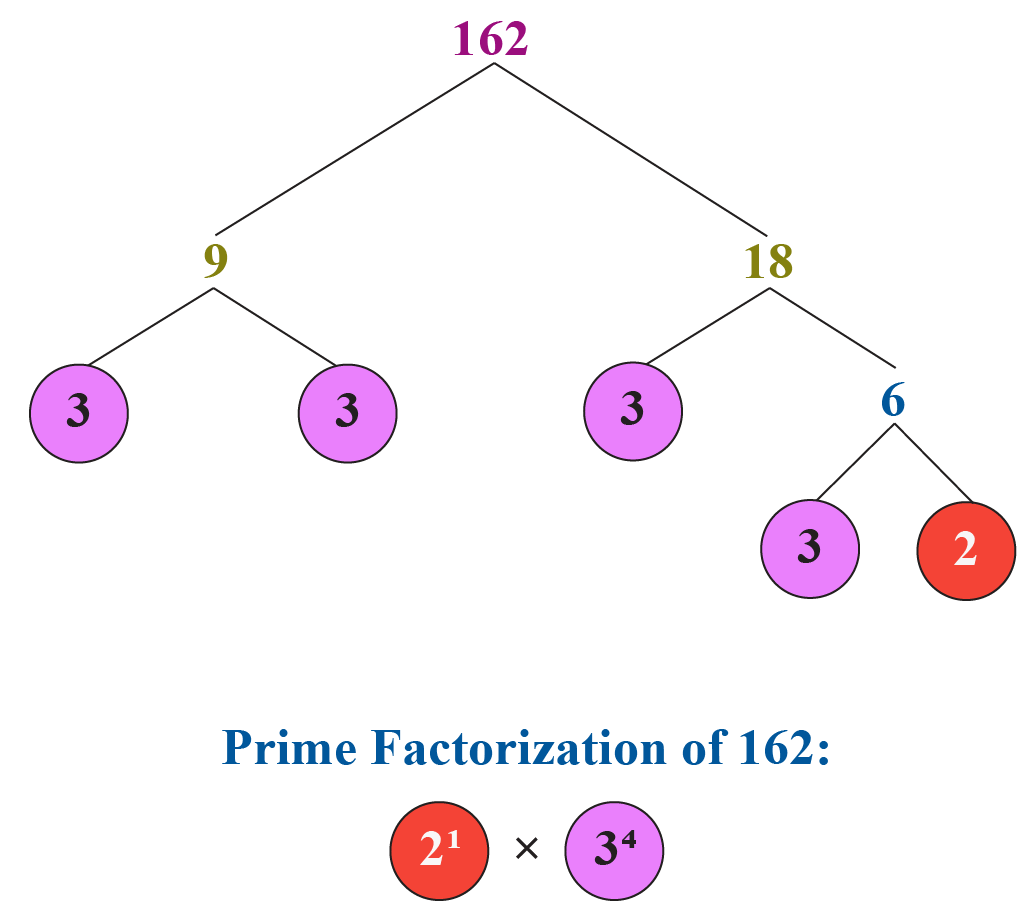The prime factorization of 180 is shown below: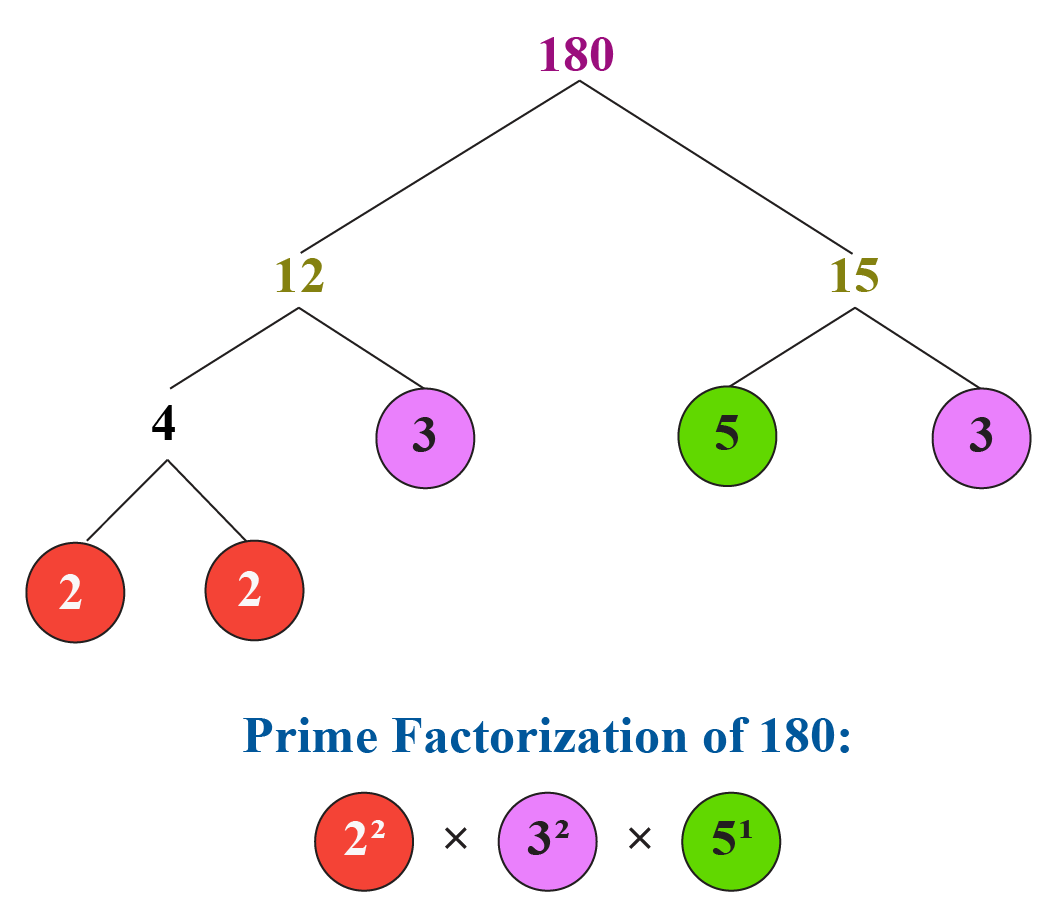Thus,

\begin{align}126&=2^{1} \times 3^{2} \times 7^{1}\\[0.3cm]162&=2^{1} \times 3^{4}\\[0.3cm]180&=2^{2} \times 3^{2} \times 5^{1}\end{align}

The HCF is the product of the smallest power of each common prime factor.

Hence,

$\text{HCF }(126,162,180) = 2^1 \times 3^2 = 18$

 $\text{HCF }(126,162,180) = 18$
 Example 3

Jenifer was given a task by her teacher to find the lowest common multiple of $$48$$ and $$72$$ using the fundamental theorem of arithmetic.

Can you help her?

Solution

We will find the prime factorizations of $$48$$ and $$72$$

The prime factorization of 48 is shown below: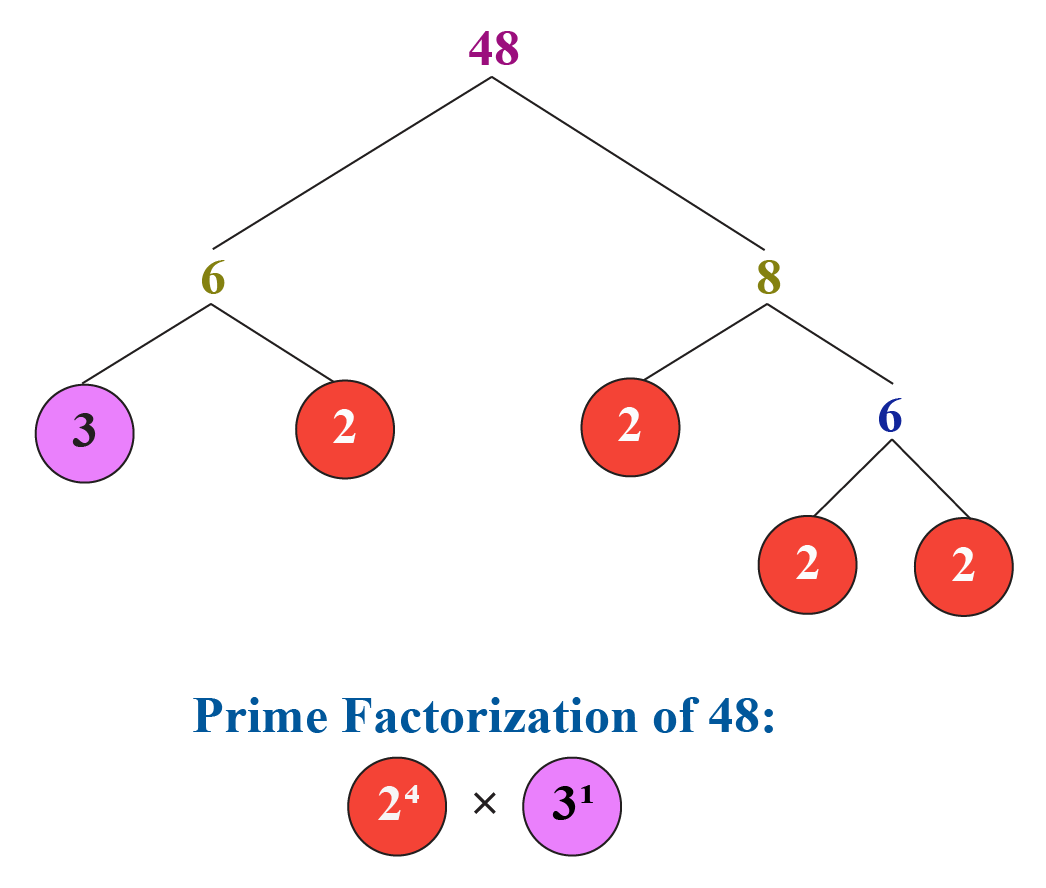The prime factorization of 72 is shown below: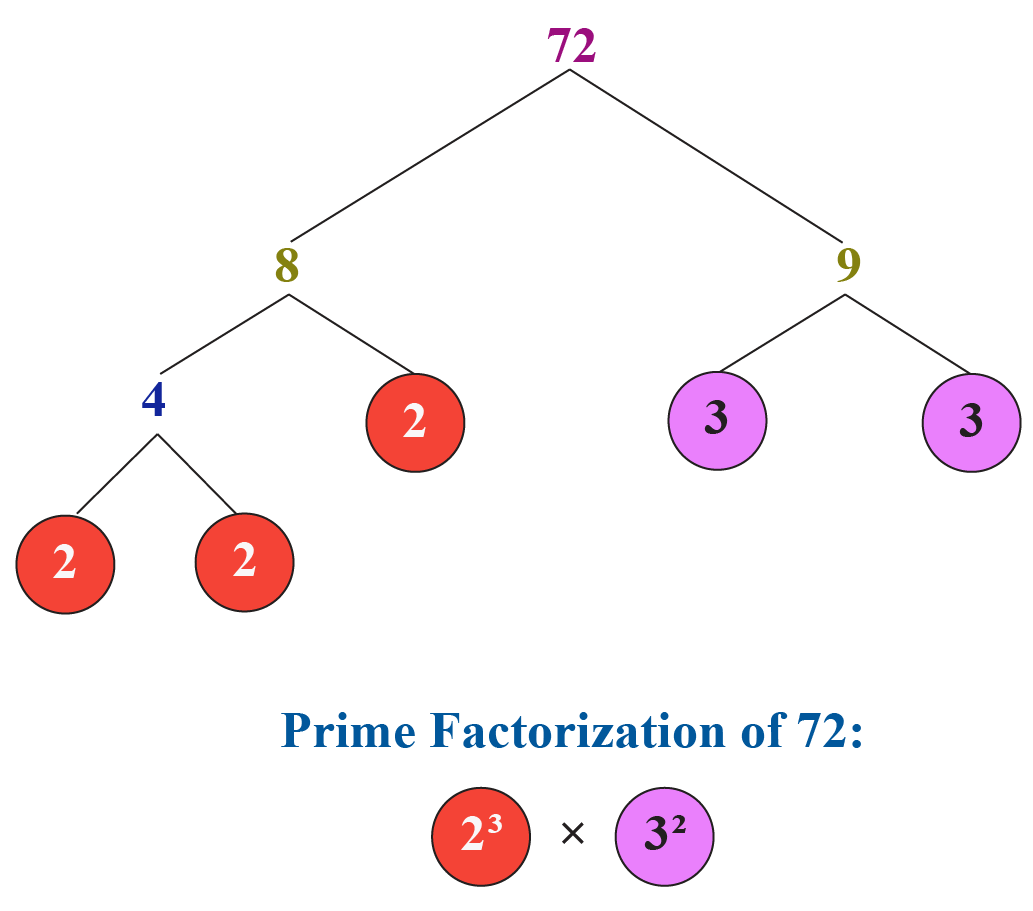The LCM or lowest common multiple of any 2 numbers is the product of the greatest power of the common prime factors.

Hence,

$\text{LCM }(48, 72) = 2^4 \times 3^2 = 144$

 Thus, $$\text{LCM }(48, 72) = 144$$
 Example 4

Jane has to prove that the prime factorization of 40 will always remain the same.

She is confused, help her prove it.Solution

Jane can use the division method and factor tree method to prove that the prime factorization of 40 will always remain the same.

Jane knows that 40 can be factored as 5 and 8

8 can further be broken down as a product of 2 and 4

5 is a prime number already.

Hence, she will show the division method and factor tree method in the following way: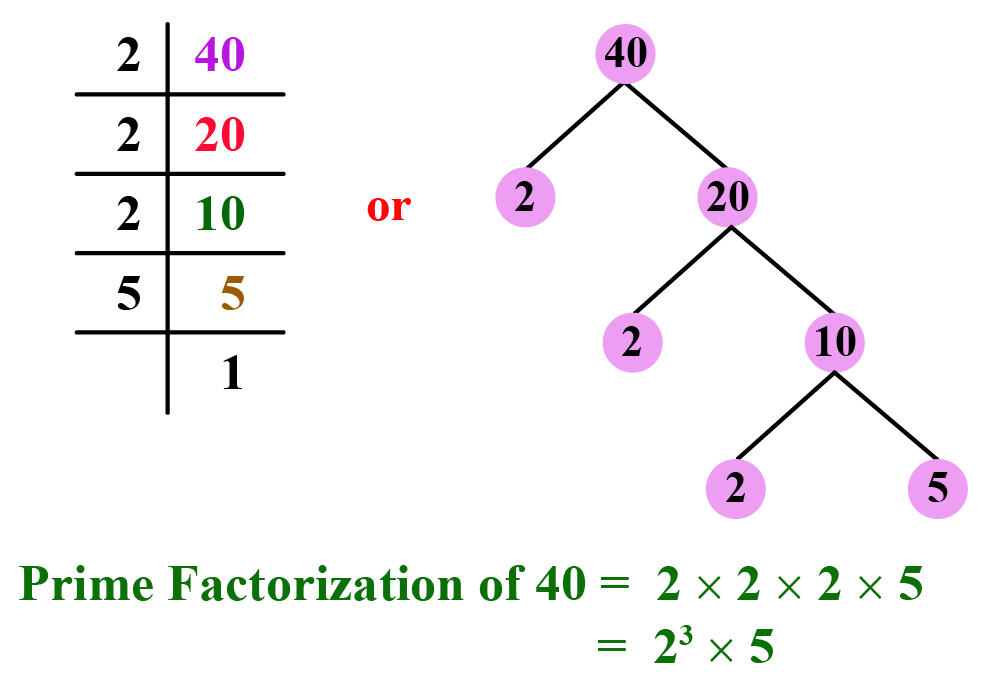$$\therefore$$ This shows that by any method of factorization, the prime factorization remains the same.

 Prime factorization for a number is unique.Challenging Questions
• How would Daniella find the prime factorization of 72 using only the factor pairs which are co-primes
• Timmothy has to split 24 students in his class into different groups with an equal number of students in each. All students cannot be in one group and each group should have more than one student. In how many ways can he form these groups?

## Interactive Questions

Here are a few activities for you to practice. Select/Type your answer and click the "Check Answer" button to see the result.

## Let's Summarize

Mini-lesson targeted the fascinating concept of prime factorization. The math journey around the prime factorization starts with what a student already knows, and goes on to creatively crafting a fresh concept in the young minds. Done in a way that not only it is relatable and easy to grasp, but also will stay with them forever. Here lies the magic with Cuemath.

At Cuemath, our team of math experts is dedicated to making learning fun for our favorite readers, the students!

Through an interactive and engaging learning-teaching-learning approach, the teachers explore all angles of a topic.

Be it worksheets, online classes, doubt sessions, or any other form of relation, it’s the logical thinking and smart learning approach that we, at Cuemath, believe in.

## 1. What is the prime factorization?

Prime factorization of any number means to represent that number as a product of prime numbers.

## 2. How to find prime factorization?

Prime factorization of any number can be found out by following two methods:

Method 1: Division method.

Method 2: Factor tree method.

## 3. What is the prime factorization of 72/36/45?

Prime factorization of 72 = $$2^3 \times 3^2$$

Prime factorization of 36 = $$2^2 \times 3^2$$

Prime factorization of 45 = $$3^2 \times 5$$

## 4. How to find LCM using prime factorization?

LCM of two numbers can be found out by first finding out the prime factors of the numbers. Then the LCM is the product of the greatest power of each common prime factor.

## 5. Where is prime factorization useful?

Prime factorization is useful in finding HCF and LCF of numbers. It is widely used in cryptography as well.

Arithmetic Integers
grade 10 | Questions Set 2
Arithmetic Integers
Arithmetic Integers
grade 10 | Questions Set 1
Arithmetic Integers